# 1st PUC Computer Science Model Question Paper 4 with Answer

Students can Download 1st PUC Computer Science Model Question Paper 4 with Answers, Karnataka 1st PUC Computer Science Model Question Papers with Answers helps you to revise the complete Karnataka State Board Syllabus and score more marks in your examinations.

## Karnataka 1st PUC Computer Science Model Question Paper 4 with Answers

Time: 3 Hours 15 Minutes
Max. Marks: 70

PART – A

I. Answer the following questions. (10 × 1 = 10)

Question 1.
Define computer memory.
The working place in a computer where all the data and instructions are stored is called memory.

Question 2.
Expand the term OMR.

Question 3.
Define algorithm.
An algorithm is a “step by step procedure to solve a given problem in a finite number of steps”.

Question 4.
Who gave the name ‘C++’?
The name C++ is given by Rick Mascitti.

Question 5.
What is an input stream?
Input stream is a sequence of characters from any input device like a keyboard to the program of a computer.

Question 6.
Give the name of a multi-branch selection statement.
The multi-branch selection statement is called as ‘switch-case’ statement.

Question 7.
What is the use of arrays?
Use of arrays reduces the creation and use of more individual variables in a program. It also helps to reduce the program length.

Question 8.
Why are arguments used in functions?
Arguments are the mechanisms that carry values from calling function to called function.Question 9.
A menu is a collection of options that perform specific activities on document.

Question 10.
What is a filter in worksheet?
A filter is an option that allows display of data of user’s choice, by hiding other nonrelevant data.

PART – B

II. Answer any Five of the following questions. (5 × 2 = 10)

Question 11.
What are the inventions of Prof. John Napier and William Oughtred?
Prof. John Napier invented a calculating device which is a set of 9 marked rods called “Napier Bones” and the device invented by William Oughtred for computations is the “slide rule”.

Question 12.
What do you mean by soft copy and hard copy?

• A soft copy is an electronic copy of data viewed on a computer’s display or transmitted as an e-mail attachment.
• A hard copy is a physical reproduction or representation of the data, such as a printed page or photograph.

Question 13.
Mention the different parts of machine language instructions.
The two parts of machine language instructions are opcodes and operand codes.

Question 14.
How are flowcharts classified?
Flowcharts are classified as system flow charts and program flowcharts.

Question 15.
Define encapsulation and polymorphism.

• The method of combining data, attributes, and methods in the same entity is called encapsulation.
• Polymorphism is a Greek word that means many shapes. In OOP Polymorphism means the ability to take on many forms. The term is applied both to objects and to operations.

Question 16.
Explain lvalue and rvalue. Give example.

• L value is the location value and the r-value is the data value of a variable, int age =16;
• L value is the location value of age and 16 is the r-value.Question 17.
What is the use of isalnumO function?
It returns true if the argument is a digit from 0 through 9 or an alphabetic character (either uppercase or lowercase) otherwise returns false.

Question 18.
What is the use of page setup option?
The page setup option is used to select the paper size and paper orientation for document printing.

PART – C

III. Answer any Five of the following questions. (5 × 3 = 15)

Question 19.
Define track, sector, and cylinder.
Tracks are concentric circles, and sectors are pie-shaped blocks on a track, where data is stored. The set of tracks of a hard disk drive which can be accessed without changing the position of the access arm are called cylinder.

Question 20.
Write the binary equivalent of decimal 28.25, octal equivalent of decimal 165 and hexadecimal equivalent of decimal 345.
The binary equivalent of decimal 28.25 is 11100.01
28(, O)=11100(2)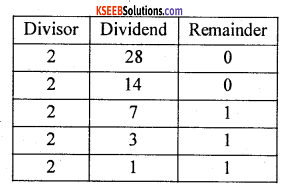The octal equivalent of decimal 165 is 245(g).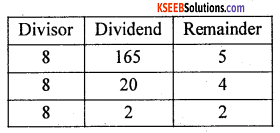The hexadecimal equivalent of decimal 345 is 159(16).Question 21.
What is a multitasking operating system? Give an example.
In multitasking operating system, several applications may be simultaneously loaded and used in memory. While the processor handles only one application at a particular time, it switches between the applications and simultaneously executes each application. For example, Windows operating system is a multitasking operating System.Question 22.
Write a flowchart to check whether a given year is a leap year or not.Question 23.
What is typecast? Mention the types of typecasting.
Typecasting is making a variable of one type, such as an int, act like another type, a char, for one single operation. The two types of typecasting are implicit conversion and explicit conversion.

Question 24.
What is cascading in I/O operations? How is cascading useful in output operations?
Cascading is a way to extract/insert multiple values from/into more than one variable using one cin/cout statement. Cascading allows the user to use cout one time but use << operator several times, to output many values.

Question 25.
Give explanation of declaration of one-dimensional array with an example.
int marks;

• Name of the array is marks.
• Type of the array is integer.
• Size of the array is 6. i.e., we can store up to 6 integer values in the array.

Question 26.
How is array of structures declared? Give an example.
The array of structure is a collection of array elements in which each element is a structure in the array.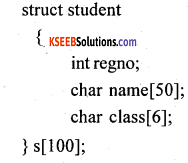PART – D

IV. Answer any Seven of the following questions. (7 × 5 = 35)

Question 27.
Explain the role of computers in the education field.
1. Students use computers for simple word processing that is, to type reports and other documents and to print out the results. This application allows students to revise and edit easily. A computer makes it much easier to redraft a sentence and produce a new printout when compared with producing a handwritten copy.

2. Encyclopedias and other reference works are available on CD-ROMs, which can be searched by the students using the computer in their classrooms or school libraries.

3. Computer-aided instruction (CAI). Interactive programs provide practice in such basic skills as spelling, math computation, and word recognition.

4. The Internet and World Wide Web is a source of information resource, that students may want to access, using their computer at school or home.

5. Computers are used in running school and college administrations, during the admission procedures, storing of official arid student records. They are also used in syllabus planning and decision-making, controlling.

6. Computers are helpful in directing aptitude tests and achievement tests, at the time of entrance exams. They also process records of salaries, examinations, schemes of examination, printing of papers and question papers, evaluation of answer sheets, mark sheets, and certificates.Question 28.
Write a note on BCD and Excess-3 code.
1. Binary Coded Decimal (BCD) code:
In this code, each decimal digit is represented by a 4- bit binary number. BCD is a way to express each of the decimal digits with a binary code. In the BCD, with four bits we can represent sixteen numbers (0000 to 1111). But in BCD code only first ten of these are used (0000 to 1001). The remaining six code combinations i.e. 1010 to 1111 are invalid in BCD.

2. Excess-3 code:
The Excess-3 code is also called an XS-3 code. It is a non-weighted code used to express decimal numbers. The Excess-3 codewords are derived from the 8421 BCD code words adding (0011)2 or (3)10 to each codeword in 8421. The excess-3 codes are obtained as follows:
Decimal Number—— >8421 BCD—> Excess – 3

Question 29.
Write a short note on structured programming.
Structured ProgrammingrThe concept was contributed by Professor Dijkstra and other colleagues made it popular.
Structured Programming deals only with logic and code and suggests making use of programming structures such as sequence, selection, iteration and modularity in programs.
Features:

1. It focuses on techniques of developing good computer programs and problem solutions.
2. The structures can be repeated within each other.
3. It is most important to consider single-entry and single-exit control in programs and structures.
4. Structured cod – is like a page, which can be read from the top to bottom without any backward references.

1. Programs are easy to write because the programming logic is well organized.
2. Programs can be functionally divided into smaller logical working units (modularity).
3. Modularity helps to easily understand the program, test and debug.
4. Easy to maintain because of single entry and single exit.
5. Eliminates the use of undisciplined controls (GOTO, BREAK, etc.,) in the program.
6. Read from top to bottom makes the code easy to read, test, debug, and maintain.

30. Write the applications of C++.

• It is a versatile language for handling very large programs
• It is suitable for virtually any programming task including development of editors, compil-ers, databases, communication systems and any complex real life application systems
• It allows us to create hierarchy-related objects, so we can build special object-oriented libraries which can be later used by many programmers
• While C++ is able to map the real-world problem properly, the C part of C++ gives the language the ability to get close to the machine – level details
• C++ programs are easily maintainable and expandable.

Question 31.
Write the syntax of if-else-if statement and the flowchart representation of if-else-if conditional structure.Question 32.
Write a program to check whether a given number is power of 2 using while state¬ment.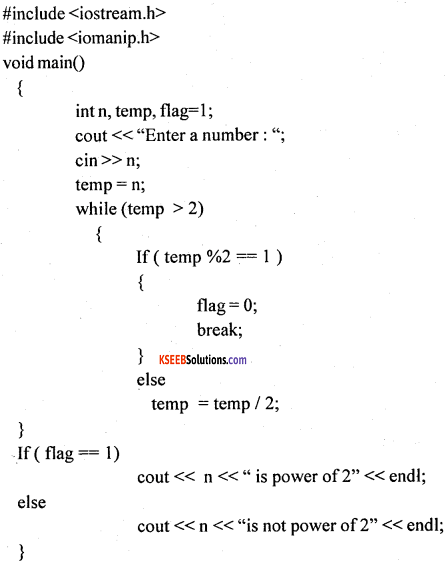Question 33.
Write a C++ program to find the sum of all the rows and sum of all the columns in a matrix.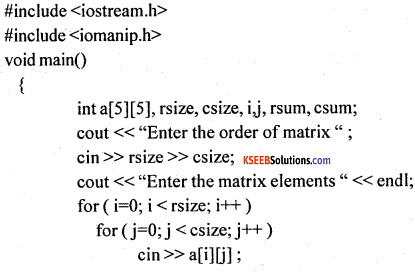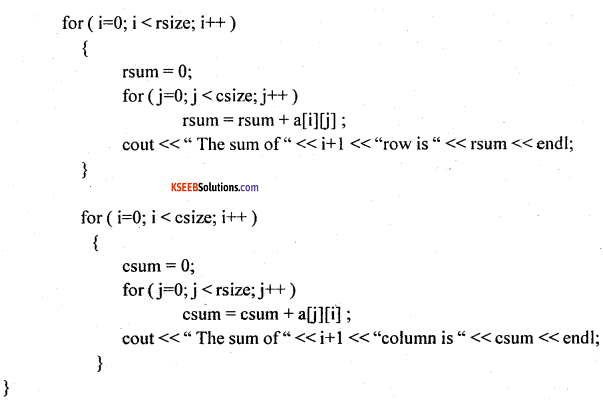Question 34.
Explain function call using pass by reference technique with an example.
Pass by reference is another way of passing parameters to the function. Pass by reference or call by reference is the method by which the addresses of the variables (actual arguments) are passed to the called function. The symbol is used to refer the addresses of the variables to the called function. The changes made to the formal parameter will change values in the actual arguments.
For example: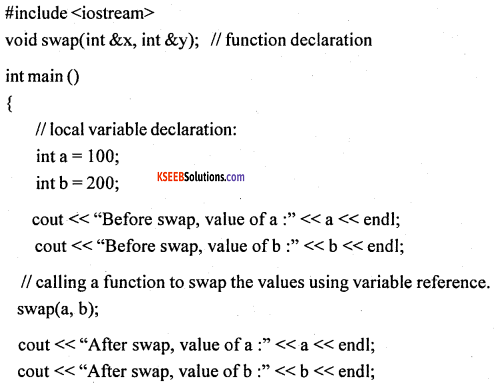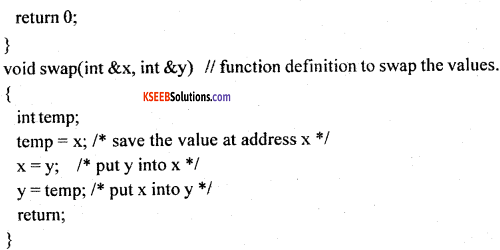Before the function call, the values of a and b were 100 and 200 respectively. After the function, though no values are returned back to the main(), shows the value of a and b as 200 and 100 respectively. This is due to call by reference in which changes made in the formal argument will affect the values in the actual argument.

Question 35.
Write a short note on data menu options.
The different options of data menu are sort, filter, validation, subtotal and pivot table options
1. Sort option:
It is used to organize the list of values either in ascending or in descending order. The custom sort allows the user apply multilevel sort to organize the data.

2. Filter option:
It is used to quickly extract certain data from the spreadsheet. It actually hides the rows or columns containing data that do not meet the filter criteria defined by the user. Excel has an AutoFilter feature that makes it very easy to extract data from the spreadsheet.

3. Validation option:
In Excel, the data validation feature helps to control what can be entered in worksheet. Users can use this option to define restrictions on what data can or should be entered in a cell. User can select range of cells and define the value that a cell can contain whether it is a whole number, or date values only, etc., User can also provide messages to define what input user expect for the cell, and instructions to help users correct any errors.

4. Subtotal option:
SUBTOTAL formula is used to find out subtotal of a given range of cells. User has to give SUBTOTAL two inputs

1.  a range of data
2. type of subtotal.
In return, SUBTOTAL will give the subtotal for that data.

5. Pivot table options:
Pivot tables in Excel are versatile reporting tools that make it easy to extract information from large tables of data without the use of formulas. They can be used to summarize, analyze, explore and present the data.Question 36.
Write a short note on formatting toolbar.
It is a toolbar that has the icons that provide the various formatting settings for cells, columns, rows and data in Excel.
For example:
it can allow the user to change the colors of rows, cells, and columns. It allows user to change fonts. It allows to set the alignment for data in cells. It allows to apply borders to cells. It allows user to change the format of numbers, like adjusting the amount of decimals they have or changing them to percentage format. All of these and other things can be done with it.

1. Format Cells Dialog Box:
For a complete list of formatting options, right-click on the highlighted cells and choose Format Cells from the shortcut menu or select Format > Cells from the menu bar.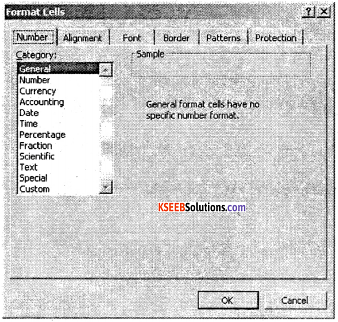2. Number tab:
The data type can be selected from the options on this tab. General is selected if the cell contains text and numbers, or a different numerical category if the cell is a number that will be included in functions or formulas. The number tab can be used to limit the number decimal places.

3. Alignment tab:
These options allow the user to change the position and alignment of the data within the cell. For example, right, left, center, vertical alignments, text wrap, etc.,

4. Font tab:
All of the font attributes are displayed in this tab including font face, size, style, and effects. For example, bold, italicize, underline, increase/decrease the font size, changing the color of font, fill, etc.,

5. Border and Pattern tabs:
These tabs allow users to add borders, shading, and background colors to a cell.

Question 37.
What is meant by webpage, web site, web browser, URL, and TCP/IP?